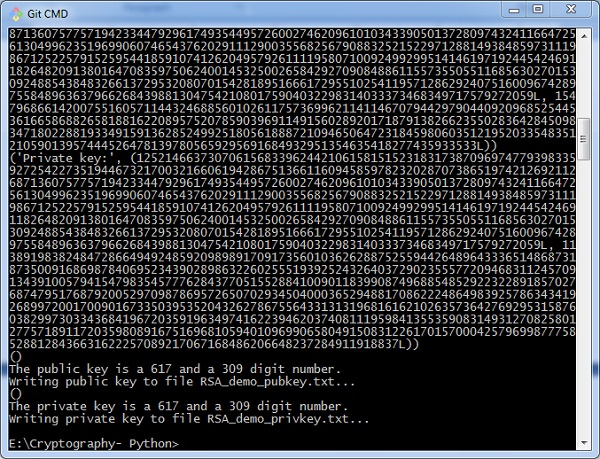# Creating RSA Keys

In this chapter, we will focus on step wise implementation of RSA algorithm using Python.

## Generating RSA keys

The following steps are involved in generating RSA keys −

• Create two large prime numbers namely p and q. The product of these numbers will be called n, where n= p*q

• Generate a random number which is relatively prime with (p-1) and (q-1). Let the number be called as e.

• Calculate the modular inverse of e. The calculated inverse will be called as d.

## Algorithms for generating RSA keys

We need two primary algorithms for generating RSA keys using Python − Cryptomath module and Rabin Miller module.

### Cryptomath Module

The source code of cryptomath module which follows all the basic implementation of RSA algorithm is as follows −

def gcd(a, b):
while a != 0:
a, b = b % a, a
return b

def findModInverse(a, m):
if gcd(a, m) != 1:
return None
u1, u2, u3 = 1, 0, a
v1, v2, v3 = 0, 1, m

while v3 != 0:
q = u3 // v3
v1, v2, v3, u1, u2, u3 = (u1 - q * v1), (u2 - q * v2), (u3 - q * v3), v1, v2, v3
return u1 % m


### RabinMiller Module

The source code of RabinMiller module which follows all the basic implementation of RSA algorithm is as follows −

import random
def rabinMiller(num):
s = num - 1
t = 0

while s % 2 == 0:
s = s // 2
t += 1
for trials in range(5):
a = random.randrange(2, num - 1)
v = pow(a, s, num)
if v != 1:
i = 0
while v != (num - 1):
if i == t - 1:
return False
else:
i = i + 1
v = (v ** 2) % num
return True
def isPrime(num):
if (num 7< 2):
return False
lowPrimes = [2, 3, 5, 7, 11, 13, 17, 19, 23, 29, 31, 37, 41, 43, 47, 53, 59, 61,
67, 71, 73, 79, 83, 89, 97, 101, 103, 107, 109, 113, 127, 131, 137, 139, 149, 151,
157, 163, 167, 173, 179, 181, 191, 193, 197, 199, 211, 223, 227, 229, 233, 239, 241,
251, 257, 263, 269, 271, 277, 281, 283, 293, 307, 311, 313,317, 331, 337, 347, 349,
353, 359, 367, 373, 379, 383, 389, 397, 401, 409, 419, 421, 431, 433, 439, 443, 449,
457, 461, 463, 467, 479, 487, 491, 499, 503, 509, 521, 523, 541, 547, 557, 563, 569,
571, 577, 587, 593, 599, 601, 607, 613, 617, 619, 631, 641, 643, 647, 653, 659, 661,
673, 677, 683, 691, 701, 709, 719, 727, 733, 739, 743, 751, 757, 761, 769, 773, 787,
797, 809, 811, 821, 823, 827, 829, 839, 853, 857, 859, 863, 877, 881, 883, 887, 907,
911, 919, 929, 937, 941, 947, 953, 967, 971, 977, 983, 991, 997]

if num in lowPrimes:
return True
for prime in lowPrimes:
if (num % prime == 0):
return False
return rabinMiller(num)
def generateLargePrime(keysize = 1024):
while True:
num = random.randrange(2**(keysize-1), 2**(keysize))
if isPrime(num):
return num


The complete code for generating RSA keys is as follows −

import random, sys, os, rabinMiller, cryptomath

def main():
makeKeyFiles('RSA_demo', 1024)

def generateKey(keySize):
# Step 1: Create two prime numbers, p and q. Calculate n = p * q.
print('Generating p prime...')
p = rabinMiller.generateLargePrime(keySize)
print('Generating q prime...')
q = rabinMiller.generateLargePrime(keySize)
n = p * q

# Step 2: Create a number e that is relatively prime to (p-1)*(q-1).
print('Generating e that is relatively prime to (p-1)*(q-1)...')
while True:
e = random.randrange(2 ** (keySize - 1), 2 ** (keySize))
if cryptomath.gcd(e, (p - 1) * (q - 1)) == 1:
break

# Step 3: Calculate d, the mod inverse of e.
print('Calculating d that is mod inverse of e...')
d = cryptomath.findModInverse(e, (p - 1) * (q - 1))
publicKey = (n, e)
privateKey = (n, d)
print('Public key:', publicKey)
print('Private key:', privateKey)
return (publicKey, privateKey)

def makeKeyFiles(name, keySize):
# Creates two files 'x_pubkey.txt' and 'x_privkey.txt'
(where x is the value in name) with the the n,e and d,e integers written in them,
# delimited by a comma.
if os.path.exists('%s_pubkey.txt' % (name)) or os.path.exists('%s_privkey.txt' % (name)):
sys.exit('WARNING: The file %s_pubkey.txt or %s_privkey.txt already exists! Use a different name or delete these files and re-run this program.' % (name, name))
publicKey, privateKey = generateKey(keySize)
print()
print('The public key is a %s and a %s digit number.' % (len(str(publicKey)), len(str(publicKey))))
print('Writing public key to file %s_pubkey.txt...' % (name))

fo = open('%s_pubkey.txt' % (name), 'w')
fo.write('%s,%s,%s' % (keySize, publicKey, publicKey))
fo.close()
print()
print('The private key is a %s and a %s digit number.' % (len(str(publicKey)), len(str(publicKey))))
print('Writing private key to file %s_privkey.txt...' % (name))

fo = open('%s_privkey.txt' % (name), 'w')
fo.write('%s,%s,%s' % (keySize, privateKey, privateKey))
fo.close()
# If makeRsaKeys.py is run (instead of imported as a module) call
# the main() function.
if __name__ == '__main__':
main()


### Output

The public key and private keys are generated and saved in the respective files as shown in the following output.